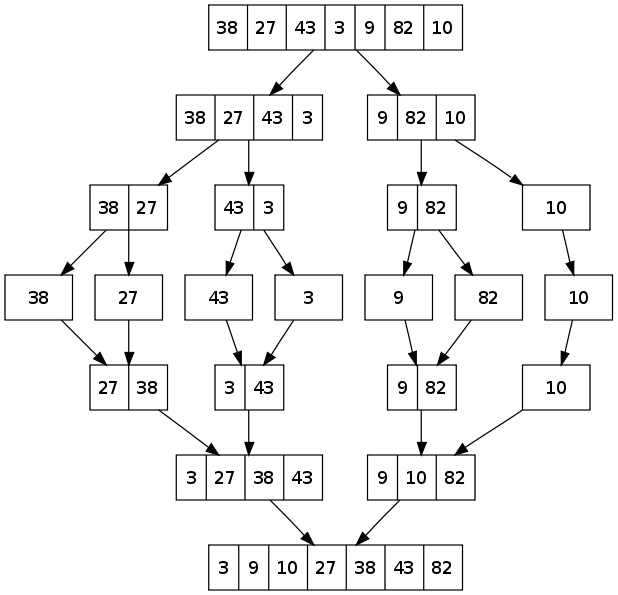# Merge Sort Basics

suggest change

Merge Sort is a divide-and-conquer algorithm. It divides the input list of length n in half successively until there are n lists of size 1. Then, pairs of lists are merged together with the smaller first element among the pair of lists being added in each step. Through successive merging and through comparison of first elements, the sorted list is built.

An example:Time Complexity: `T(n) = 2T(n/2) + Θ(n)`

The above recurrence can be solved either using Recurrence Tree method or Master method. It falls in case II of Master Method and solution of the recurrence is `Θ(nLogn)`. Time complexity of Merge Sort is `Θ(nLogn)` in all 3 cases (worst, average and best) as merge sort always divides the array in two halves and take linear time to merge two halves.

Auxiliary Space: `O(n)`